# Venn diagram in ggplot2

Chun-Hui Gao

## Venn diagram with `ggVennDiagram`

`ggVennDiagram` allows creating Venn diagrams based on ggplot2. You need to pass a list of vectors containing your data to the `ggVennDiagram` function as in the following example. Note that character vectors will be transformed into numeric.

``````# install.packages("ggVennDiagram")
library(ggVennDiagram)

# List of items
x <- list(A = 1:5, B = 2:7)

# 2D Venn diagram
ggVennDiagram(x)``````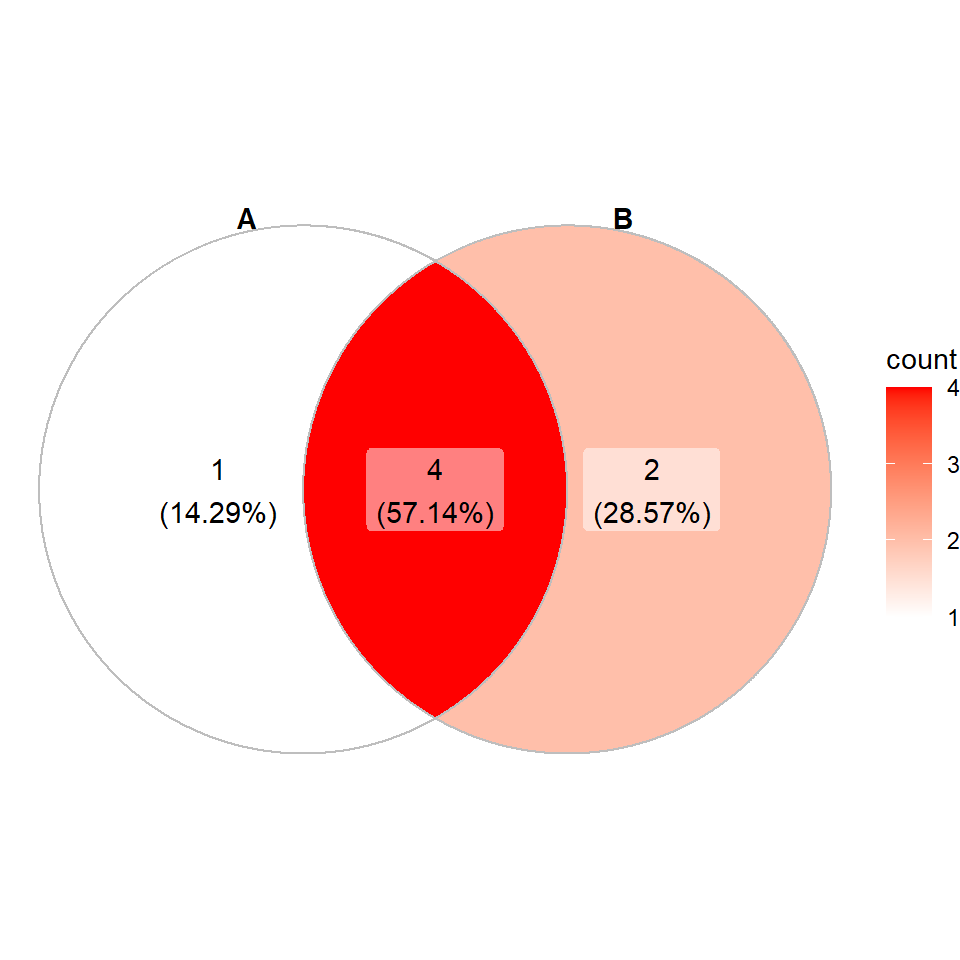3D Venn diagram

If you pass a list with three elements you will get some Venn diagram like the following.

``````# install.packages("ggVennDiagram")
library(ggVennDiagram)

# List of items
x <- list(A = 1:5, B = 2:7, C = 5:10)

# 3D Venn diagram
ggVennDiagram(x)``````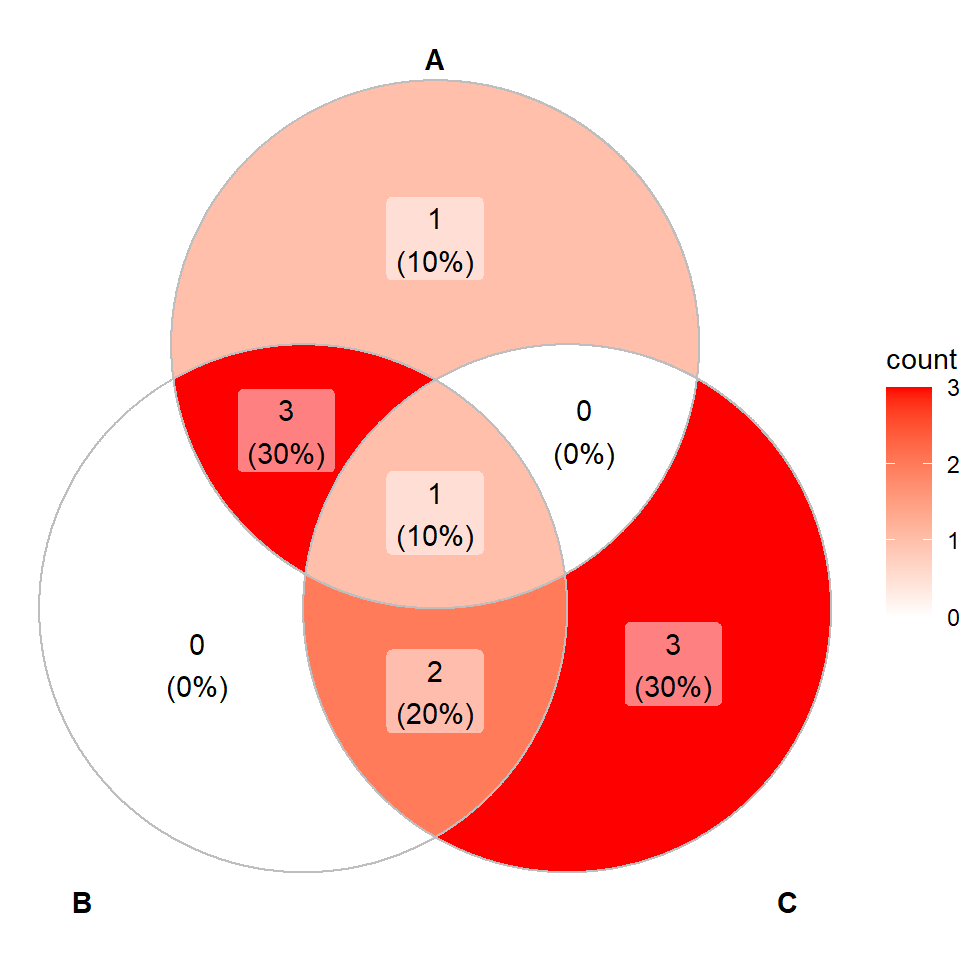4D Venn diagram

You can also pass a list with up to four different elements. If you need to add more refer to the documentation.

``````# install.packages("ggVennDiagram")
library(ggVennDiagram)

# List of items
x <- list(A = 1:5, B = 2:7, C = 5:10, D = 8:15)

# 4D Venn diagram
ggVennDiagram(x)``````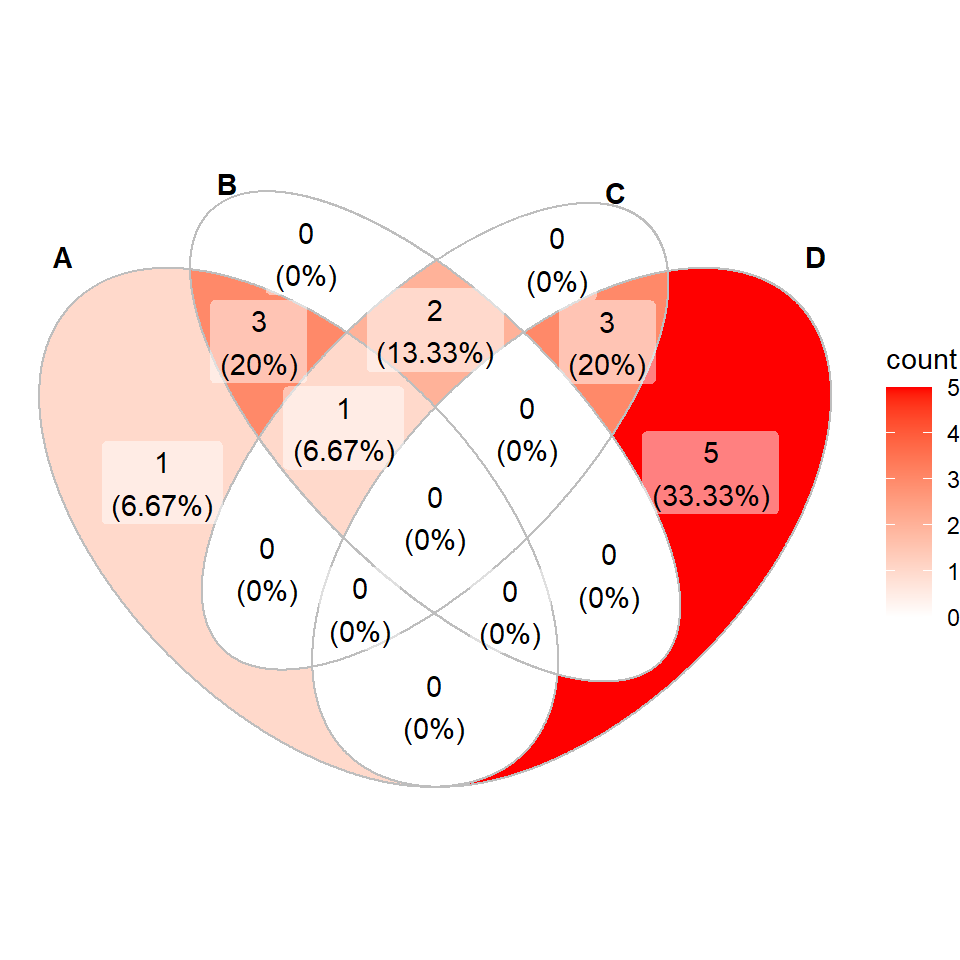### Changing the colors of the diagram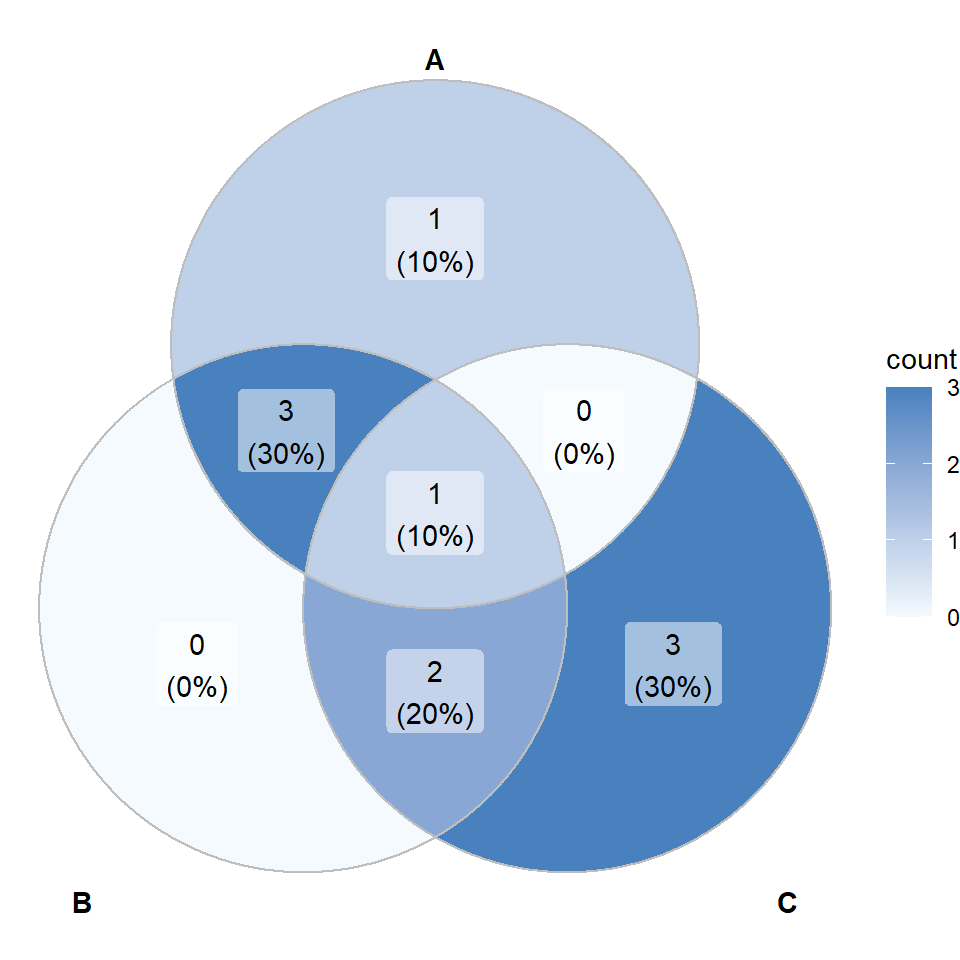As `ggVennDiagram` is based on ggplot2 you can add more layers or override the ones existing. In order to change the colors use `scale_fill_gradient` as in the following example.

``````# install.packages("ggVennDiagram")
library(ggVennDiagram)
# install.packages("ggplot2")
library(ggplot2)

# List of items
x <- list(A = 1:5, B = 2:7, C = 5:10)

# Venn diagram with custom colors
ggVennDiagram(x) +
scale_fill_gradient(low = "#F4FAFE", high = "#4981BF")``````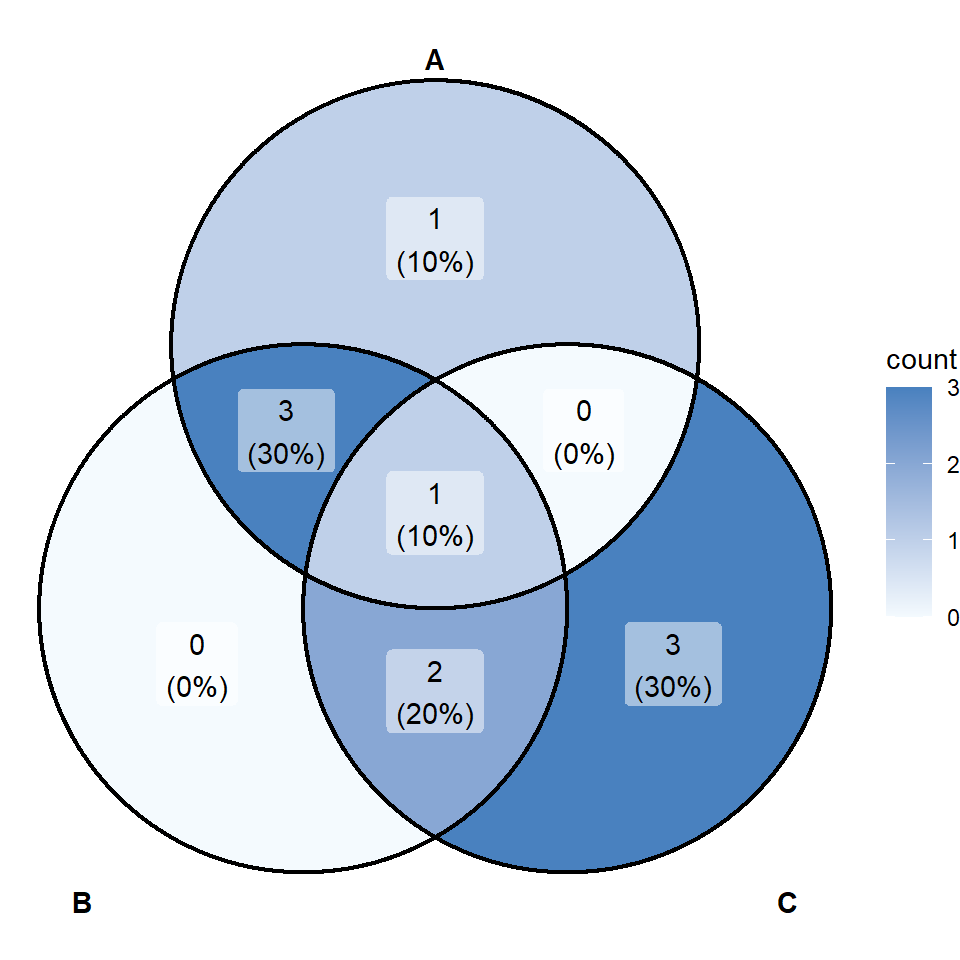The border can also be customized but making use of `color` argument. The line width and line style can be changed with `lwd` and `lty`, respectively.

``````# install.packages("ggVennDiagram")
library(ggVennDiagram)
# install.packages("ggplot2")
library(ggplot2)

# List of items
x <- list(A = 1:5, B = 2:7, C = 5:10)

# Venn diagram with custom border
ggVennDiagram(x, color = "black", lwd = 0.8, lty = 1) +
scale_fill_gradient(low = "#F4FAFE", high = "#4981BF")``````

### Labels and group names

Group names

By default, the function uses the names of the list to set the category names, but you can override them with `category.names`.

``````# install.packages("ggVennDiagram")
library(ggVennDiagram)

# List of items
x <- list(A = 1:5, B = 2:7, C = 5:10)

# Venn diagram with custom category names
ggVennDiagram(x, category.names = c("Group 1",
"Group 2",
"Group 3"))``````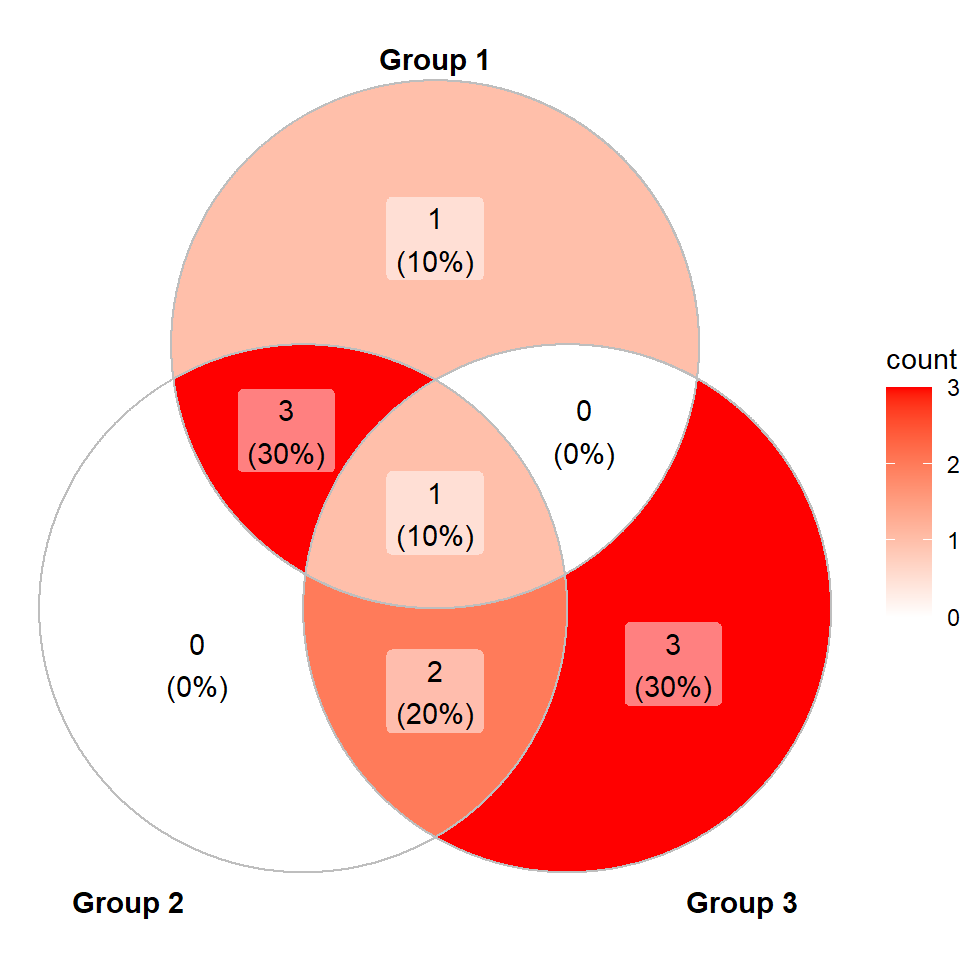Labels with percentages

Labels display percentages and the count of values. Set `label = "percent"` to only show percentages.

``````# install.packages("ggVennDiagram")
library(ggVennDiagram)

# List of items
x <- list(A = 1:5, B = 2:7, C = 5:10)

# Venn diagram with percentages
ggVennDiagram(x,
label = "percent")``````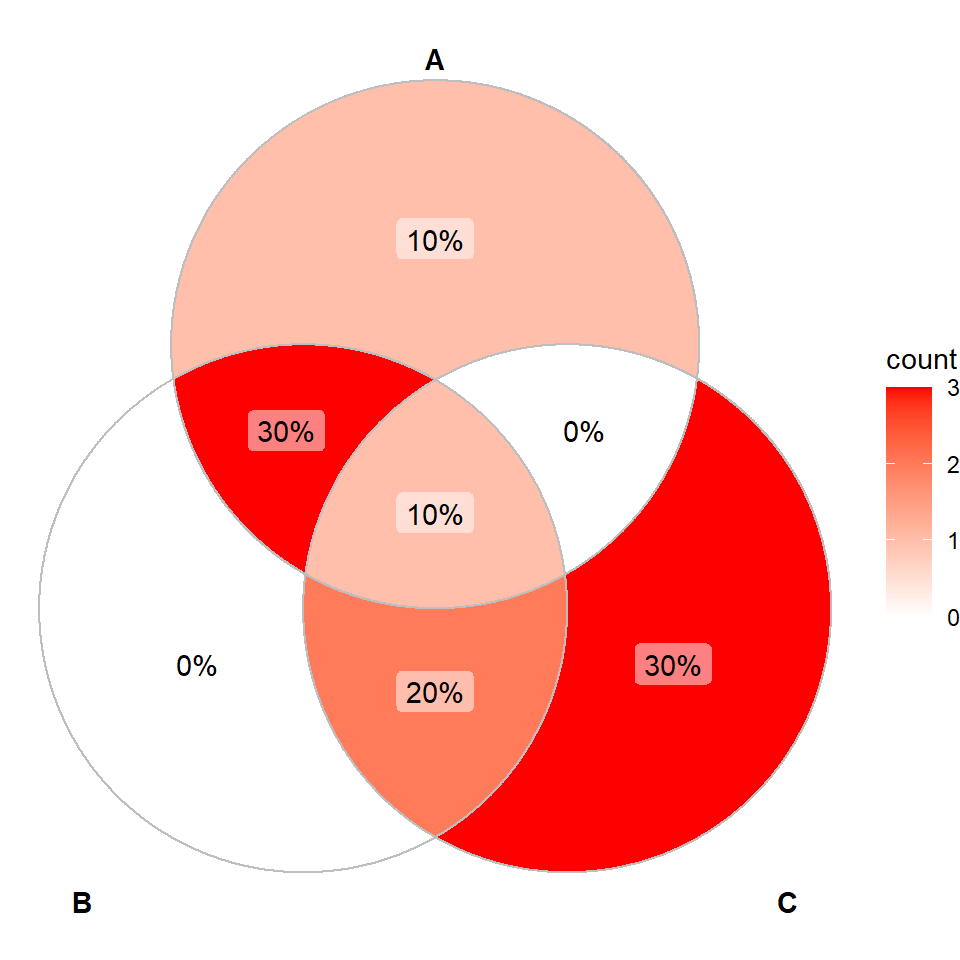Labels with count

If you prefer showing only the count set `label = "count"`.

``````# install.packages("ggVennDiagram")
library(ggVennDiagram)

# List of items
x <- list(A = 1:5, B = 2:7, C = 5:10)

# Venn diagram with count labels
ggVennDiagram(x,
label = "count")``````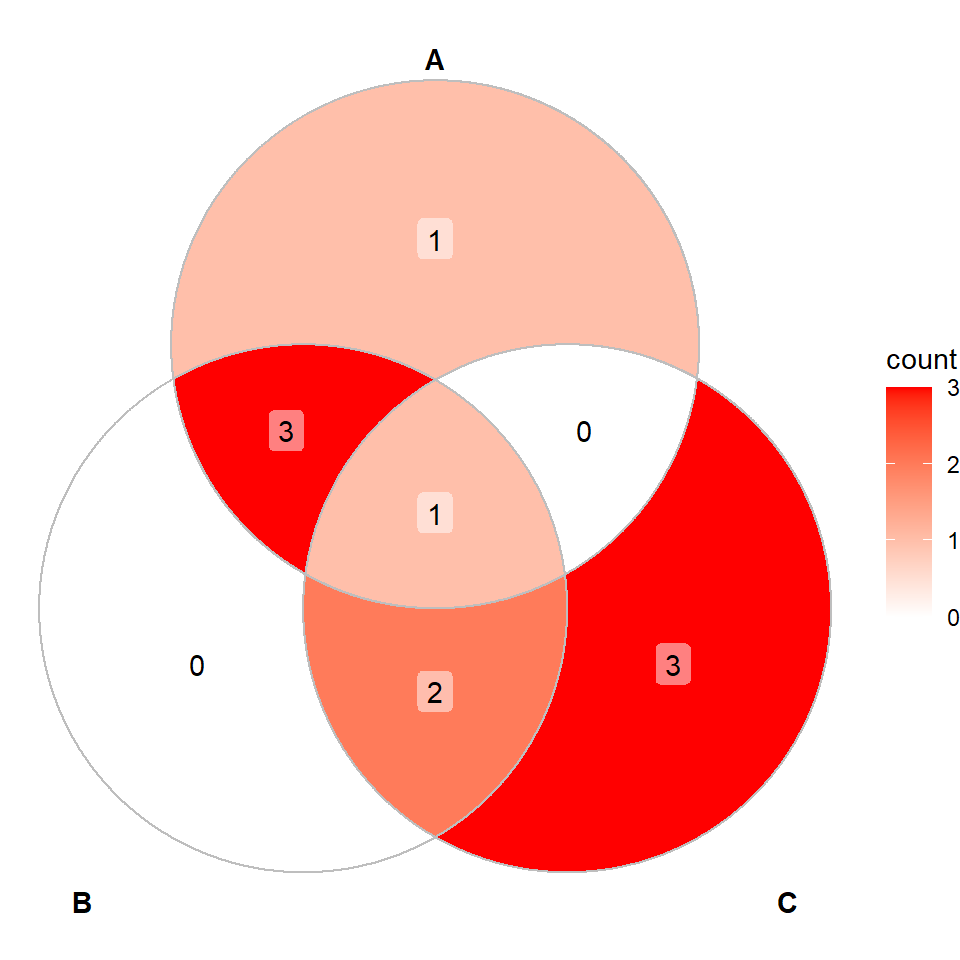Remove the labels

It is possible to remove all the labels just setting `label = NULL`.

``````# install.packages("ggVennDiagram")
library(ggVennDiagram)

# List of items
x <- list(A = 1:5, B = 2:7, C = 5:10)

# Venn diagram without labels
ggVennDiagram(x,
label = NULL)``````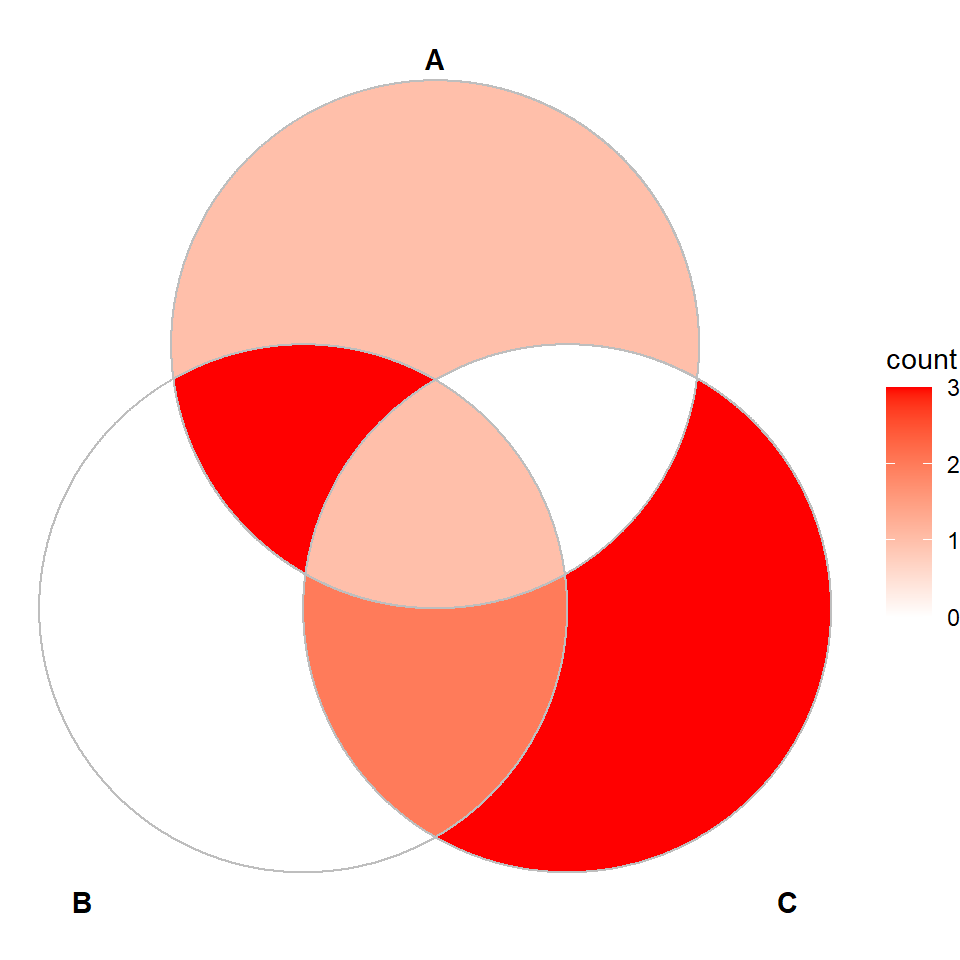Labels transparency

If you install the development version of the package from GitHub there is an additional argument that allows modifying the transparency of the background of the labels.

``````# install.packages("devtools")
# devtools::install_github("gaospecial/ggVennDiagram")
library(ggVennDiagram)

# List of items
x <- list(A = 1:5, B = 2:7, C = 5:10)

# Labels transparency
ggVennDiagram(x,
label_alpha = 0)``````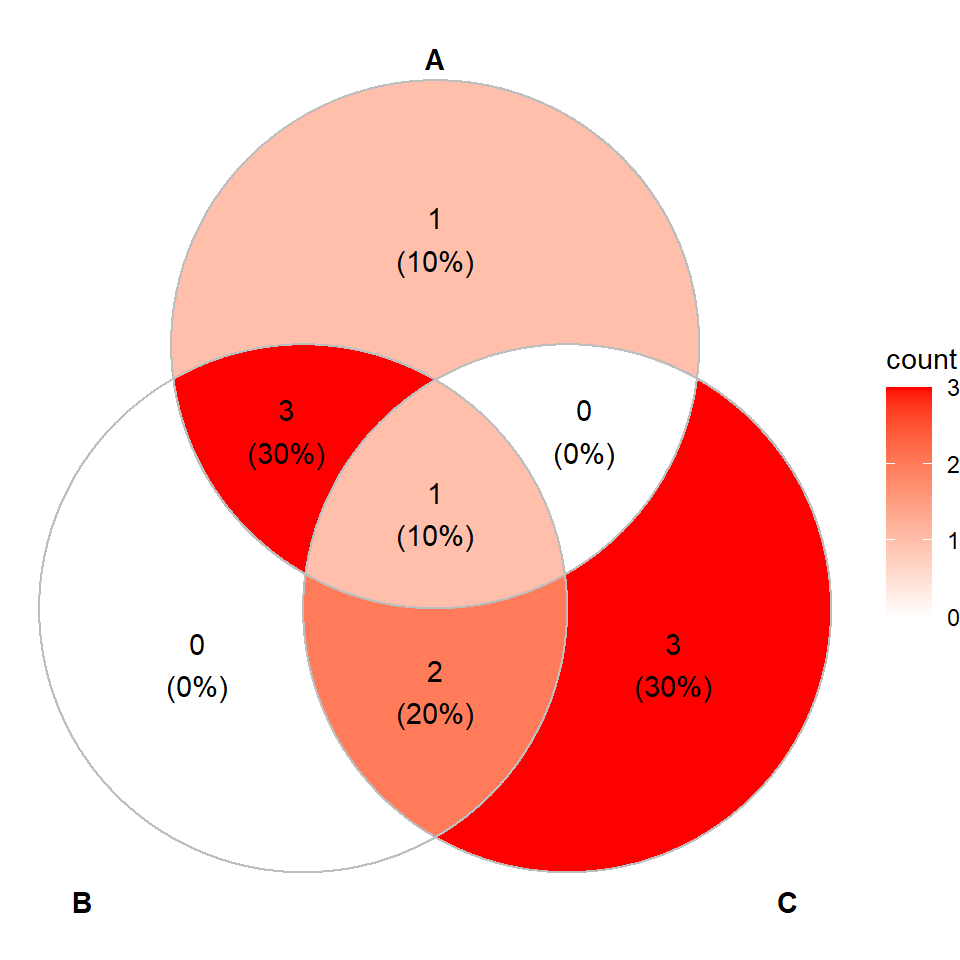### Remove or customize the legend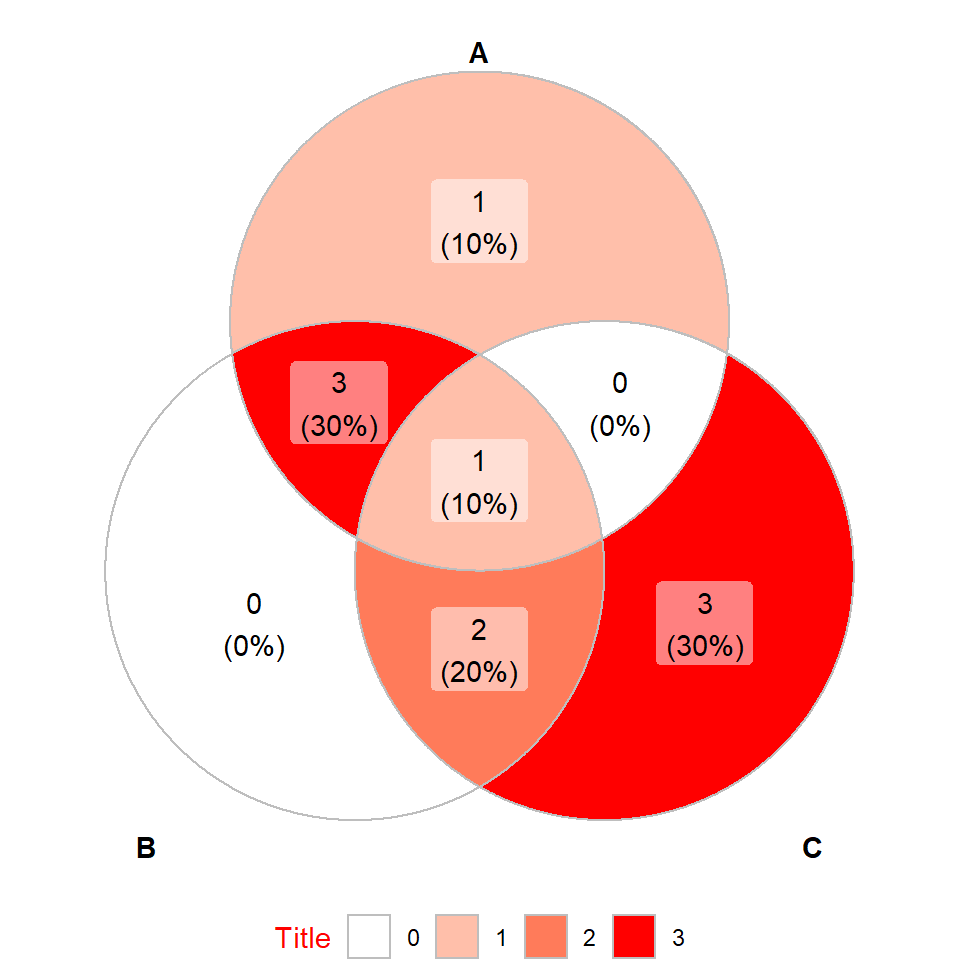The legend of the Venn diagrams can be customized adding more layers to the plot. In the following example we are modifying the legend title and the legend position.

``````# install.packages("ggVennDiagram")
library(ggVennDiagram)
# install.packages("ggplot2")
library(ggplot2)

# List of items
x <- list(A = 1:5, B = 2:7, C = 5:10)

# Venn diagram with custom legend
ggVennDiagram(x) +
guides(fill = guide_legend(title = "Title")) +
theme(legend.title = element_text(color = "red"),
legend.position = "bottom")``````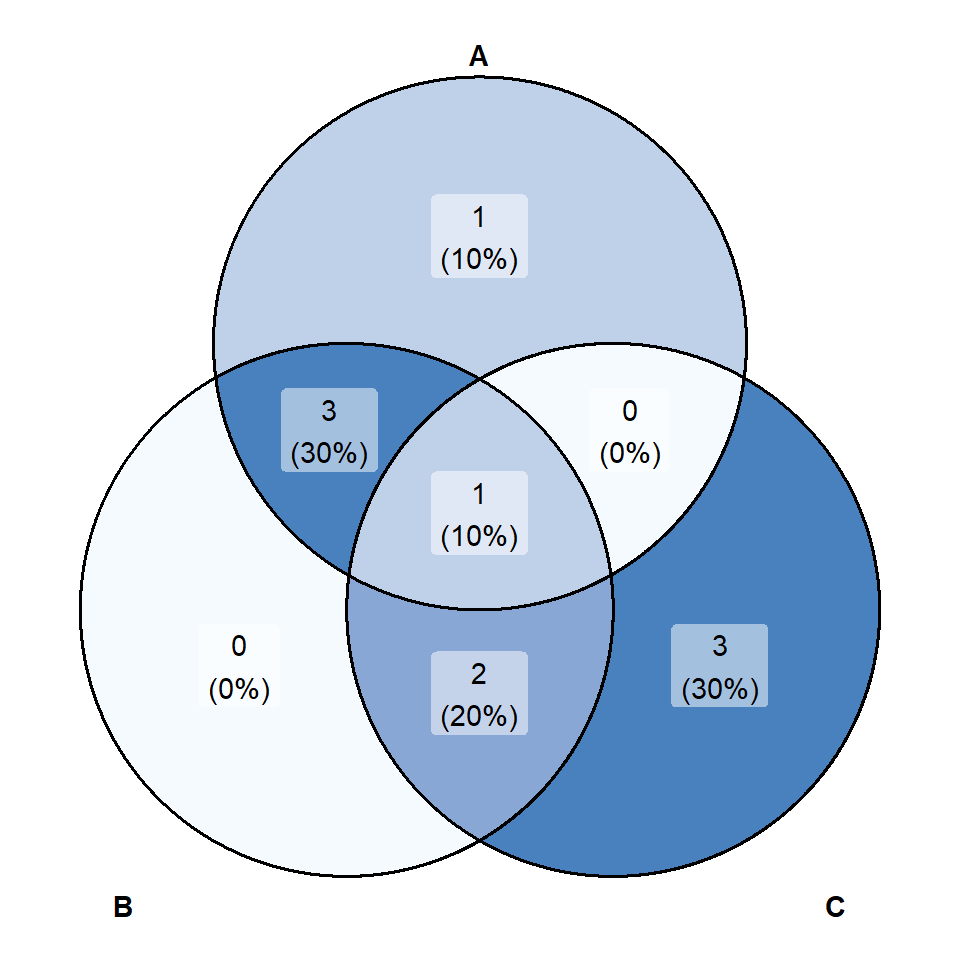It is possible to remove the legend of the diagram just setting its position to `"none"`.

``````# install.packages("ggVennDiagram")
library(ggVennDiagram)
# install.packages("ggplot2")
library(ggplot2)

# List of items
x <- list(A = 1:5, B = 2:7, C = 5:10)

# Venn diagram without legend
ggVennDiagram(x, color = 1, lwd = 0.7) +
scale_fill_gradient(low = "#F4FAFE", high = "#4981BF") +
theme(legend.position = "none")``````